# Tree

A tree is a connected, acyclic, undirected graph. Trees are named for their resemblance to the eponymous tall, woody plants, as these are also connected and acyclic (a tree is in one piece, but its branches never form cycles). A collection of disjoint trees is known as a forest.

## Characterization

For a simple graph, any two of these three statements, taken together, imply the third (proof):

• The graph is connected.
• The graph is acyclic.
• The number of vertices in the graph is exactly one more than the number of edges.

This implies that when two of these conditions are known to hold, the graph is definitely a tree, and that all trees satisfy all three conditions (with the exception of the empty tree, which contains no nodes at all, and is sometimes considered not to be a valid tree anyway).

Additionally, the statement that there is exactly one path between any pair of vertices is also equivalent to the statement that the graph is a tree.

All trees are planar, but not all planar graphs are trees.

## Anatomy

Some trees are rooted, that is, they have one particular vertex designated the root. Others are unrooted, perhaps because no significance can be attached to singling out one vertex to be a root. Here are examples, that serve to introduce and explain terminology:

• In an organic tree, there are several points at which the trunk and its branches divide. Let these, along with the ground, and the endpoints of the smallest branches, be vertices, with the vertex at the ground being labelled the root, for obvious reasons, and let the trunk and branches be the edges. The significance of the rooting is that the trunk and all its branches are considered to emanate from the root (which makes sense given how trees actually grow). The vertices corresponding to the endpoints of the smallest branches, which always have degree one, are called leaves, in strict analogy. The distance from the root node to any other node is sometimes called that node's height, again in strict analogy, but, due to the practice in computer science of drawing trees upside-down, it is more commonly called the depth. The maximum height of any node in the tree is known as the tree's height.
• The family tree consisting of all descendants of an individual: This, too, is named for its resemblance to an organic tree, and it is no surprise that it can be placed into analogy with a graph-theoretic tree as well. Let each person in the family tree be a vertex and let there be an edge between two vertices if one of the corresponding individuals is a child of the other. Label the vertex corresponding to the aforementioned individual as the root vertex. Then, all adjacent vertices represent that individual's child, and, in strict analogy, these nodes are said to be children of the root node. In general, in a rooted tree, if two nodes are adjacent, the one further away from the root is considered to be a child of the one closer, and the one closer is called the parent of the one further away. (A node will always have exactly one parent, unless it is the root.) Furthermore, given some node$u$, the node itself, its children, its children's children, and so on, are called the node's descendants; the term proper descendant is sometimes taken to mean the same but excluding$u$ itself. Likewise,$u$,$u$'s parent,$u$'s parent's parent, and so on, are called ancestors (with the term proper ancestor being defined analogously). The root is ancestral to all nodes.
• In the tree of life, each vertex represents a species and edges represent evolution of one species into another. The root of this tree is some universal common ancestor of life on Earth, and the leaves of this tree are either extant species or species that have gone extinct without radiating or transitioning to a newer species.
• A corporate hierarchy can theoretically be represented by a rooted tree, where each employee is a vertex and a vertex's children are those vertices corresponding to the employees managed by subordinates, and the vertex corresponding to the CEO (or equivalent) is the root of the tree. Hierarchies in general are easily representable by trees.
• Given some initially disconnected cities, we might wish to build some roads between pairs of cities such that there is exactly one path between any pair of cities; having fewer than one would imply that some cities are not reachable from each other, and having more than one would introduce redundancies. So we build roads in such a way that the cities are vertices and the roads edges of a tree. This is an example of an unrooted tree because there is no specific reason why any node should be labelled the root.
• When a pair of parallel, closely spaced wooden boards with some nails hammered into them is immersed in a solution of water, soap, and glycerin, and then removed, a soap film will be formed that connects all the nails together without forming any cycles . This gives what is called a Steiner tree. Again, this is an unrooted tree, as it makes no sense to label any particular vertex the root.

The distinction between rooted and unrooted trees is somewhat artificial; we can always convert a rooted tree to an unrooted tree by forgetting which node is the root, and we may sometimes wish, in algorithms, to regard an unrooted tree as rooted by arbitrarily choosing a root.

Note that in a rooted tree, the root is not considered to be a leaf even when it has degree one (unless it actually has no children, which occurs only when it is the only node in the tree). On the other hand, in an unrooted tree, any node with degree one is considered a leaf.

Any connected subgraph of a tree is itself a tree and is called a subtree of that tree, but in a rooted tree, the term is usually used in a restricted sense to denote a node$u$ plus all of its descendants; this is referred to as the subtree rooted at$u$. (The subtree rooted at the root is the tree itself.) We shall use the term in this restricted sense throughout this article. A subtree can correspond to, in the examples above, everything emanating from a specific branch of an organic tree, or all the descendants of one particular individual, or a clade (monophyletic taxon), or all the employees subject to a particular employee's authority.

## Tree traversal

Tree traversal is the act of visiting every vertex of a tree. Traversing a tree produces an ordering of the tree's vertices. If a tree has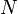$N$ nodes, then there are$N!$ different orderings, but two are particularly important: preorder and postorder. In a preorder traversal, we perform a depth-first search starting from the tree's root, and a node is considered to be visited as soon as it is first pushed onto the stack. A postorder traversal is similar, but now a node is considered to be visited only after all its children have been visited. (Leaf nodes are still considered visited as soon as they are pushed on the stack, as they have no children.) In some trees, an order is already imposed on the children of any given vertex; that is, a node with multiple children has a well-defined first child, second child, and so on. In these case, preorder and postorder traversals always visit a node's children in order, and are unique for a given rooted tree.

## Binary and k-ary trees

A binary tree is a rooted tree in which every vertex has at most two children, called left and right. The subtree rooted at a node's left child is called the left subtree, with right subtree defined analogously. Binary trees are of special interest because of their ability to organize data, as in binary search trees and binary heaps.

A binary tree is regarded as having an infinite number of levels, only finitely many of which are usually filled. A level consists of all nodes of a given depth. The zeroth level consists of only the root; the first level consists of the root's children, that is, the potential nodes of depth one, of which there are at most two; the second level consists of all of the root's potential grandchildren, that is, the potential nodes of depth two, of which there are at most four, and so on; in general, level$h$ can contain up to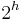$2^h$ nodes. If a binary tree has height$h$, then the maximum number of nodes it might contain is then$1 + 2 + 4 + ... + 2^h = 2^{h+1}-1$. Likewise, a binary tree containing$N$ nodes has height at least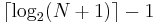$\lceil \log_2(N+1) \rceil - 1$.

There is some variation in the relationship between the terms leaf, internal node, and external node. Here are three possibilities:

• All nodes in the binary tree are considered internal nodes, with the leaves being the internal nodes that have zero children. If an internal node has fewer than two children, the children it might have had are designated external nodes. The external nodes are not actually part of the tree.
• All nodes in the binary tree are considered internal nodes. If an internal node has fewer than two children, the children it might have had are designated external nodes, also known as leaves.
• All nodes in the binary tree must have either zero or two children. The nodes that have two children are called internal nodes, whereas those that have zero children are called leaves or external nodes.

These three conventions are really only different ways of labelling nodes, but the fact that all three are in use can be confusing. The reason why different conventions exist is probably that, in some applications, only the internal nodes are meaningful, whereas in others, the external nodes are sentinels, and in others still, data are stored only in leaves. The first convention is the one used in ACSL Short Questions and the one we shall use in this article.

It can be proven inductively that the number of external nodes in a tree is always one more than the number of internal nodes. The internal path length of a binary tree is the sum of the lengths of the paths from the root to all other internal nodes; it is not hard to see that this is also equal to the sum of the depths of all the internal nodes in the tree. The external path length is analogously defined. It can be proven inductively that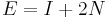$E = I + 2N$, where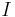$I$ is the internal path length,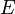$E$ is the external path length, and$N$ is the number of internal nodes.

A binary tree is said to be complete when levels 0 through$h-1$ are completely filled, and all nodes on level$h$ are as far to the left as possible. A complete binary tree will always have the minimum height possible. A binary tree is said to be balanced when all external nodes have similar height; if the heights of any two external nodes differ by at most one, it is said to be perfectly balanced. Balanced trees are useful for searching efficiently.

A k-ary tree is a rooted tree in which every vertex has at most$k$ children. Similar terminology applies.

### Inorder traversal

In addition to preorder and postorder, a binary tree has an inorder traversal, in which a node is considered to be visited after its left subtree, if any, has been completely explored, but before its right child has been pushed onto the stack. The inorder traversal is unique for a given binary tree, and inorder traversal of a binary search tree always gives a sorted list.

## Tree data structures

The following data structures are based on rooted trees, often binary trees:

• Binary search tree: The label of a node is always at least as large as that of its left child, if any, and smaller than that of its right child, if any. Binary search trees enable efficient implementations of dictionaries.
• Binary heap: A complete binary tree in which the label of each node is always at least as large as that of both of its children, if any.
• The binomial heap is a variation on the binary heap that preserves its efficiency while also enabling heaps to be efficiently merged.
• The Fibonacci heap is a variation on the binary heap that, in amortized analysis, is even faster than the binomial heap in most operations.
• Segment tree, range tree, or interval tree: A binary tree representing an array in which leaf nodes represent individual elements of the array and the datum stored in each non-leaf node is an associative binary function of the data stored in its children. Segment trees enable efficient dynamic computation of associative functions on segments of the array.
• Binary indexed tree (BIT) or Fenwick tree: Similar to a segment tree, but enables efficient dynamic computation of associative functions only on prefixes of the array. Nevertheless, if the function and the elements of the array form a group structure, inclusion-exclusion allows efficient queries on non-initial segments too.
• Parse tree: The tree that results from parsing a string. A parse tree has symbols, that is, atomic sequences of characters, in its leaves, and traversing the tree left-to-right and concatenating all the symbols gives the original string. Each subtree of a parse tree represents a substring and non-leaf nodes carry information about the syntactic relationship between the substrings represented by the subtrees rooted at their immediate children.
• Abstract syntax tree: An abstract syntax tree (AST) is similar to a parse tree, but it carries abstract information about the parsed string rather than concrete information. Its leaves are then elementary concepts and the internal nodes encode logical rather than syntactic relationships (which might be called meaning).
• A kd-tree stores$k$-dimensional points in a way that enables efficient solution of nearest-neighbor search and other problems.
• A trie or prefix tree is a tree in which every node contains a character and every path from root to internal node spells out a prefix. Tries are useful for organizing strings.
• A suffix tree is similar to a prefix tree, but paths now spell out suffixes rather than prefixes. Suffix trees enable efficient solutions to many important string problems, but are very difficult to construct.
• The link/cut tree, a data structure devised by Sleator and Tarjan, supports efficient queries on a dynamic weighted forest, and gives fast solutions to the maximum flow problem.

## Tree problems

The following are problems involving trees, or problems involving graphs that have more efficient or elegant solutions when trees are involved.

• Minimum spanning tree problem: A tree$T$ is said to span a graph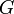$G$ when$T$ is a subgraph of$G$ that contains all of$G$'s vertices. The weight of a tree is the sum of the weights of its edges. Find a spanning tree of minimum weight.
• Lowest common ancestor (LCA) problem: Given a pair of nodes in a tree, efficiently determine their lowest common ancestor, that is, the deepest node that is an ancestor of both given nodes. A variety of algorithms exist for this important problem.
• It is easy to find the distance between any pair of nodes in a tree, weighted or unweighted, because there is only one path to consider, which may be found using depth-first search or breadth-first search.
• To find the diameter of a tree, pick any starting vertex$u$, find the vertex$v$ furthest away from$u$ using DFS or BFS (breaking ties arbitrarily), and then find the vertex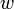$w$ furthest away from$v$. The distance between$v$ and$w$ is the tree's diameter.
• In the dynamic distance query problem, we wish to be able to efficiently determine the distances between pairs of nodes in a tree, but we also want to be able to change the weights of edges. This problem is solved using the heavy-light decomposition.
• The maximum matching problem, minimum vertex cover problem, minimum edge cover problem, and maximum independent set problem all admit simple dynamic programming solutions when the graph involved is a tree.